# Functional Programming in Pattern-Match-Oriented Programming Style <Programming> 2020 Research Paper

9 Apr 2021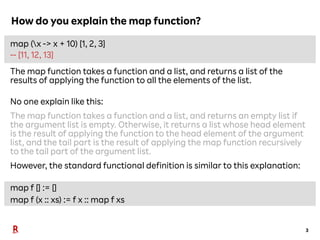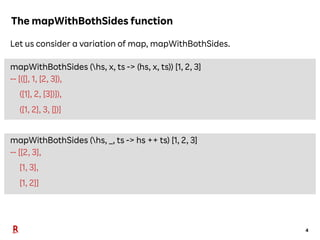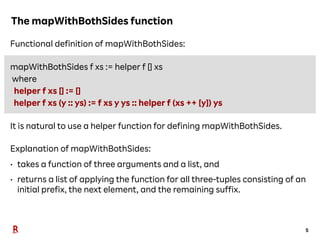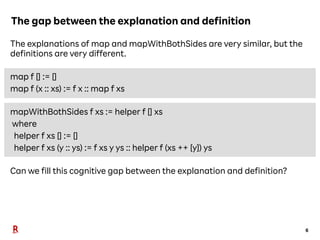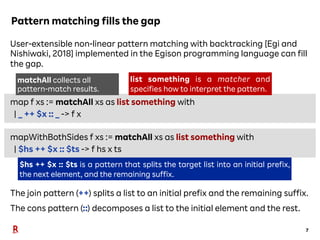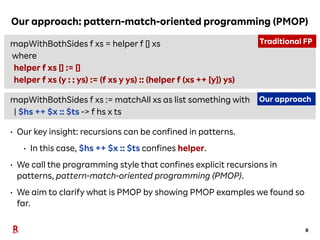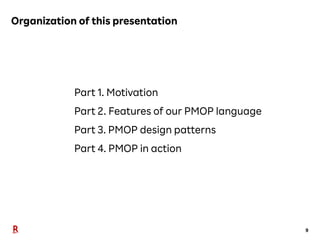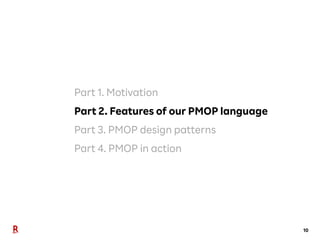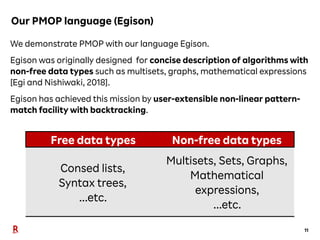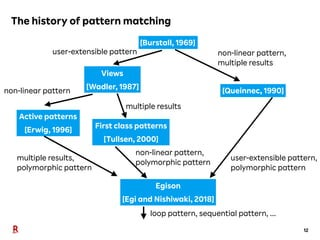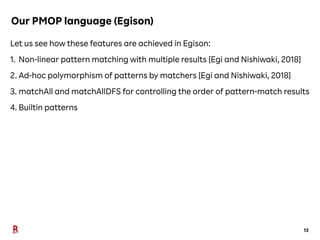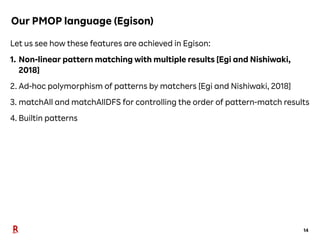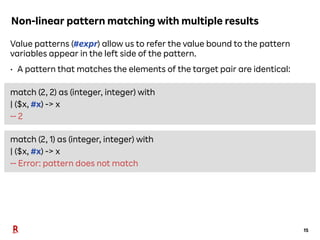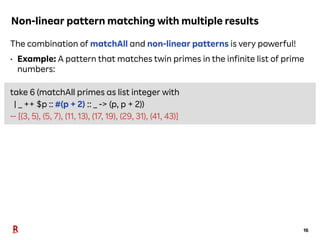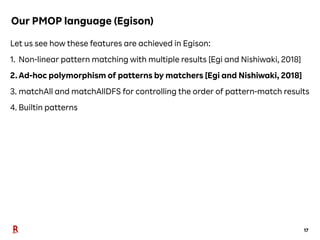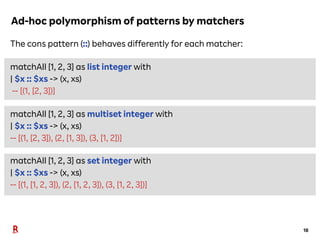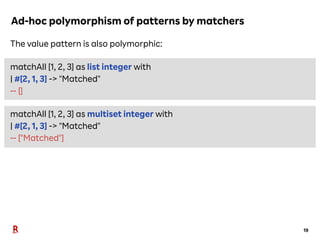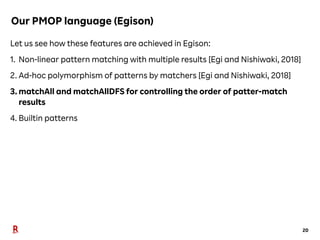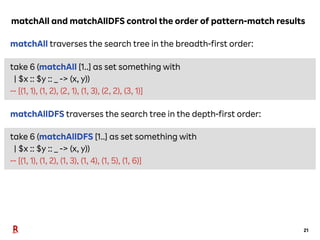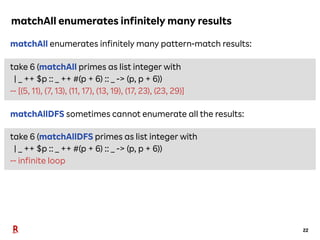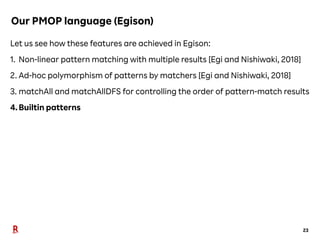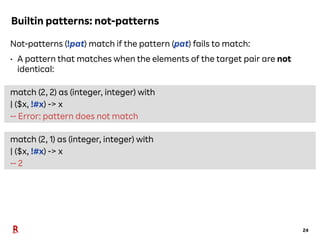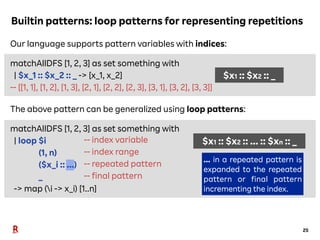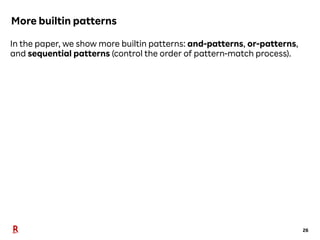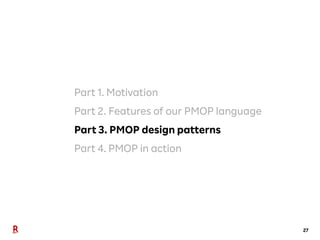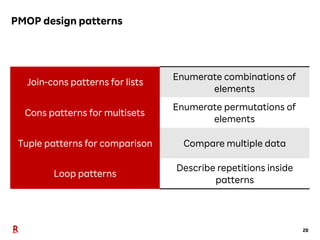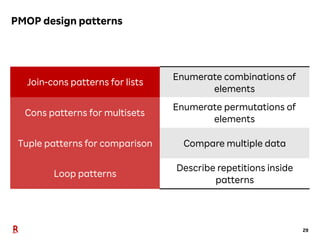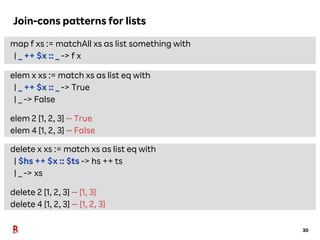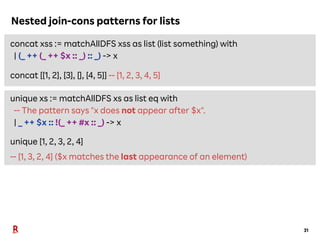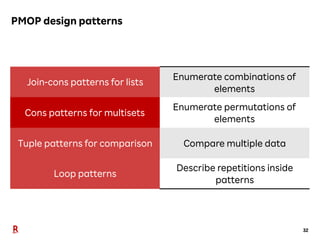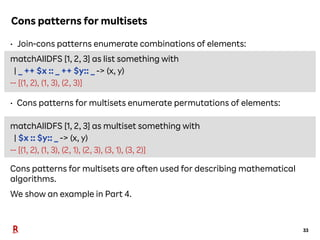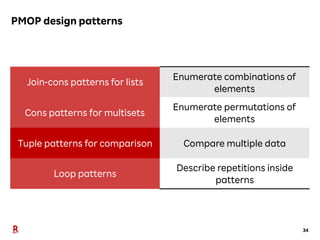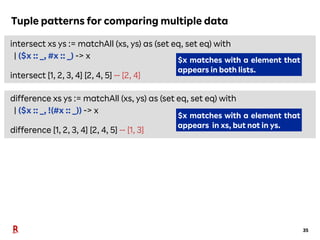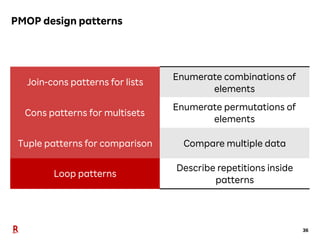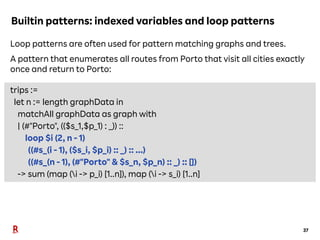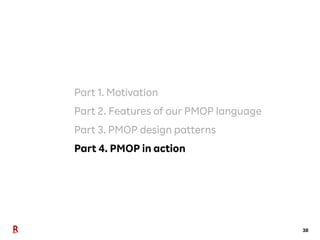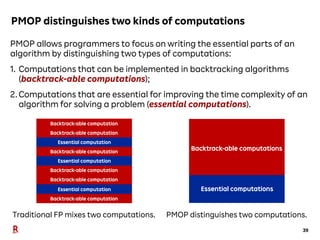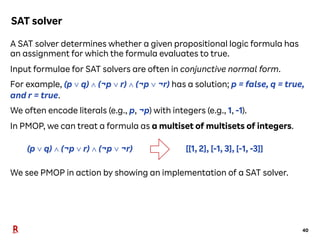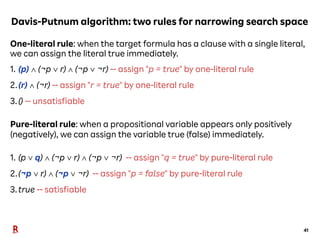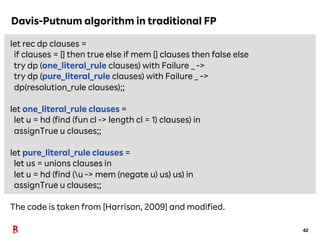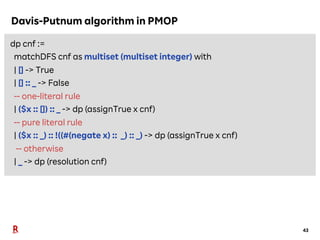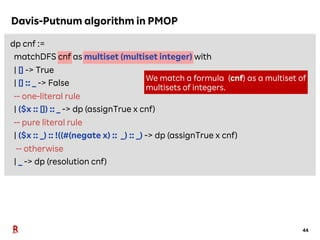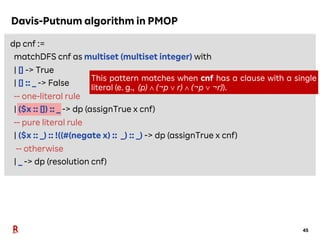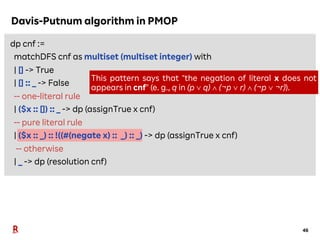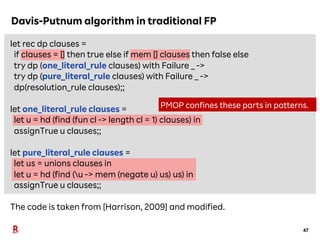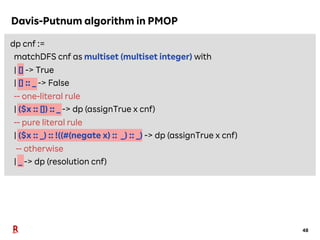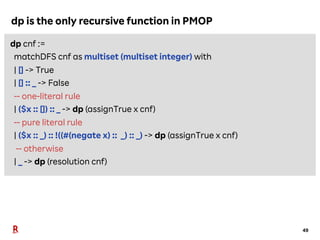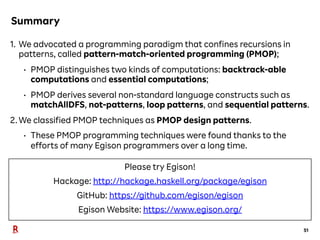1 sur 52

### Functional Programming in Pattern-Match-Oriented Programming Style <Programming> 2020 Research Paper

• 1. Functional Programming in Pattern- Match-Oriented Programming Style <Programming> 2020 Research Paper Mar 22-26th, 2021 Satoshi Egi (Rakuten Institute of Technology, Rakuten, Inc.) Yuichi Nishiwaki (The University of Tokyo)
• 3. 3 How do you explain the map function? map (x -> x + 10) [1, 2, 3] -- [11, 12, 13] The map function takes a function and a list, and returns a list of the results of applying the function to all the elements of the list. No one explain like this: The map function takes a function and a list, and returns an empty list if the argument list is empty. Otherwise, it returns a list whose head element is the result of applying the function to the head element of the argument list, and the tail part is the result of applying the map function recursively to the tail part of the argument list. However, the standard functional definition is similar to this explanation: map f [] := [] map f (x :: xs) := f x :: map f xs
• 4. The mapWithBothSides function 4 Let us consider a variation of map, mapWithBothSides. mapWithBothSides (hs, x, ts -> (hs, x, ts)) [1, 2, 3] -- [([], 1, [2, 3]), (, 2, )]), ([1, 2], 3, [])] mapWithBothSides (hs, _, ts -> hs ++ ts) [1, 2, 3] -- [[2, 3], [1, 3], [1, 2]]
• 5. The mapWithBothSides function 5 Functional definition of mapWithBothSides: mapWithBothSides f xs := helper f [] xs where helper f xs [] := [] helper f xs (y :: ys) := f xs y ys :: helper f (xs ++ [y]) ys It is natural to use a helper function for defining mapWithBothSides. Explanation of mapWithBothSides: • takes a function of three arguments and a list, and • returns a list of applying the function for all three-tuples consisting of an initial prefix, the next element, and the remaining suffix.
• 6. The gap between the explanation and definition 6 The explanations of map and mapWithBothSides are very similar, but the definitions are very different. map f [] := [] map f (x :: xs) := f x :: map f xs mapWithBothSides f xs := helper f [] xs where helper f xs [] := [] helper f xs (y :: ys) := f xs y ys :: helper f (xs ++ [y]) ys Can we fill this cognitive gap between the explanation and definition?
• 7. Pattern matching fills the gap 7 User-extensible non-linear pattern matching with backtracking [Egi and Nishiwaki, 2018] implemented in the Egison programming language can fill the gap. map f xs := matchAll xs as list something with | _ ++ \$x :: _ -> f x mapWithBothSides f xs := matchAll xs as list something with | \$hs ++ \$x :: \$ts -> f hs x ts The join pattern (++) splits a list to an initial prefix and the remaining suffix. The cons pattern (::) decomposes a list to the initial element and the rest. matchAll collects all pattern-match results. list something is a matcher and specifies how to interpret the pattern. \$hs ++ \$x :: \$ts is a pattern that splits the target list into an initial prefix, the next element, and the remaining suffix.
• 8. Our approach: pattern-match-oriented programming (PMOP) 8 mapWithBothSides f xs = helper f [] xs where helper f xs [] := [] helper f xs (y : : ys) := (f xs y ys) :: (helper f (xs ++ [y]) ys) mapWithBothSides f xs := matchAll xs as list something with | \$hs ++ \$x :: \$ts -> f hs x ts • Our key insight: recursions can be confined in patterns. • In this case, \$hs ++ \$x :: \$ts confines helper. • We call the programming style that confines explicit recursions in patterns, pattern-match-oriented programming (PMOP). • We aim to clarify what is PMOP by showing PMOP examples we found so far. Traditional FP Our approach
• 9. Organization of this presentation 9 Part 1. Motivation Part 2. Features of our PMOP language Part 3. PMOP design patterns Part 4. PMOP in action
• 10. 10 Part 1. Motivation Part 2. Features of our PMOP language Part 3. PMOP design patterns Part 4. PMOP in action
• 11. Our PMOP language (Egison) 11 We demonstrate PMOP with our language Egison. Egison was originally designed for concise description of algorithms with non-free data types such as multisets, graphs, mathematical expressions [Egi and Nishiwaki, 2018]. Egison has achieved this mission by user-extensible non-linear pattern- match facility with backtracking. Free data types Non-free data types Consed lists, Syntax trees, ...etc. Multisets, Sets, Graphs, Mathematical expressions, ...etc.
• 12. The history of pattern matching 12 [Burstall, 1969] Views [Wadler, 1987] Active patterns [Erwig, 1996] First class patterns [Tullsen, 2000] [Queinnec, 1990] Egison [Egi and Nishiwaki, 2018] non-linear pattern, multiple results user-extensible pattern, polymorphic pattern user-extensible pattern non-linear pattern multiple results multiple results, polymorphic pattern non-linear pattern, polymorphic pattern loop pattern, sequential pattern, ...
• 13. Our PMOP language (Egison) 13 Let us see how these features are achieved in Egison: 1. Non-linear pattern matching with multiple results [Egi and Nishiwaki, 2018] 2. Ad-hoc polymorphism of patterns by matchers [Egi and Nishiwaki, 2018] 3. matchAll and matchAllDFS for controlling the order of pattern-match results 4. Builtin patterns
• 14. Our PMOP language (Egison) 14 Let us see how these features are achieved in Egison: 1. Non-linear pattern matching with multiple results [Egi and Nishiwaki, 2018] 2. Ad-hoc polymorphism of patterns by matchers [Egi and Nishiwaki, 2018] 3. matchAll and matchAllDFS for controlling the order of pattern-match results 4. Builtin patterns
• 15. Non-linear pattern matching with multiple results 15 Value patterns (#expr) allow us to refer the value bound to the pattern variables appear in the left side of the pattern. • A pattern that matches the elements of the target pair are identical: match (2, 2) as (integer, integer) with | (\$x, #x) -> x -- 2 match (2, 1) as (integer, integer) with | (\$x, #x) -> x -- Error: pattern does not match
• 16. Non-linear pattern matching with multiple results 16 The combination of matchAll and non-linear patterns is very powerful! • Example: A pattern that matches twin primes in the infinite list of prime numbers: take 6 (matchAll primes as list integer with | _ ++ \$p :: #(p + 2) :: _ -> (p, p + 2)) -- [(3, 5), (5, 7), (11, 13), (17, 19), (29, 31), (41, 43)]
• 17. Our PMOP language (Egison) 17 Let us see how these features are achieved in Egison: 1. Non-linear pattern matching with multiple results [Egi and Nishiwaki, 2018] 2.Ad-hoc polymorphism of patterns by matchers [Egi and Nishiwaki, 2018] 3. matchAll and matchAllDFS for controlling the order of pattern-match results 4. Builtin patterns
• 18. Ad-hoc polymorphism of patterns by matchers 18 The cons pattern (::) behaves differently for each matcher: matchAll [1, 2, 3] as list integer with | \$x :: \$xs -> (x, xs) -- [(1, [2, 3])] matchAll [1, 2, 3] as multiset integer with | \$x :: \$xs -> (x, xs) -- [(1, [2, 3]), (2, [1, 3]), (3, [1, 2])] matchAll [1, 2, 3] as set integer with | \$x :: \$xs -> (x, xs) -- [(1, [1, 2, 3]), (2, [1, 2, 3]), (3, [1, 2, 3])]
• 19. Ad-hoc polymorphism of patterns by matchers 19 The value pattern is also polymorphic: matchAll [1, 2, 3] as list integer with | #[2, 1, 3] -> "Matched" -- [] matchAll [1, 2, 3] as multiset integer with | #[2, 1, 3] -> "Matched" -- ["Matched"]
• 20. Our PMOP language (Egison) 20 Let us see how these features are achieved in Egison: 1. Non-linear pattern matching with multiple results [Egi and Nishiwaki, 2018] 2. Ad-hoc polymorphism of patterns by matchers [Egi and Nishiwaki, 2018] 3. matchAll and matchAllDFS for controlling the order of patter-match results 4. Builtin patterns
• 21. matchAll and matchAllDFS control the order of pattern-match results 21 matchAll traverses the search tree in the breadth-first order: take 6 (matchAll [1..] as set something with | \$x :: \$y :: _ -> (x, y)) -- [(1, 1), (1, 2), (2, 1), (1, 3), (2, 2), (3, 1)] matchAllDFS traverses the search tree in the depth-first order: take 6 (matchAllDFS [1..] as set something with | \$x :: \$y :: _ -> (x, y)) -- [(1, 1), (1, 2), (1, 3), (1, 4), (1, 5), (1, 6)]
• 22. matchAll enumerates infinitely many results 22 matchAll enumerates infinitely many pattern-match results: take 6 (matchAll primes as list integer with | _ ++ \$p :: _ ++ #(p + 6) :: _ -> (p, p + 6)) -- [(5, 11), (7, 13), (11, 17), (13, 19), (17, 23), (23, 29)] matchAllDFS sometimes cannot enumerate all the results: take 6 (matchAllDFS primes as list integer with | _ ++ \$p :: _ ++ #(p + 6) :: _ -> (p, p + 6)) -- infinite loop
• 23. Our PMOP language (Egison) 23 Let us see how these features are achieved in Egison: 1. Non-linear pattern matching with multiple results [Egi and Nishiwaki, 2018] 2. Ad-hoc polymorphism of patterns by matchers [Egi and Nishiwaki, 2018] 3. matchAll and matchAllDFS for controlling the order of pattern-match results 4.Builtin patterns
• 24. Builtin patterns: not-patterns 24 Not-patterns (!pat) match if the pattern (pat) fails to match: • A pattern that matches when the elements of the target pair are not identical: match (2, 2) as (integer, integer) with | (\$x, !#x) -> x -- Error: pattern does not match match (2, 1) as (integer, integer) with | (\$x, !#x) -> x -- 2
• 25. Our language supports pattern variables with indices: matchAllDFS [1, 2, 3] as set something with | \$x_1 :: \$x_2 :: _ -> [x_1, x_2] -- [[1, 1], [1, 2], [1, 3], [2, 1], [2, 2], [2, 3], [3, 1], [3, 2], [3, 3]] The above pattern can be generalized using loop patterns: matchAllDFS [1, 2, 3] as set something with | loop \$i (1, n) (\$x_i :: ...) _ -> map (i -> x_i) [1..n] Builtin patterns: loop patterns for representing repetitions 25 -- index variable -- index range -- repeated pattern -- final pattern ... in a repeated pattern is expanded to the repeated pattern or final pattern incrementing the index. \$x1 :: \$x2 :: ... :: \$xn :: _ \$x1 :: \$x2 :: _
• 26. More builtin patterns 26 In the paper, we show more builtin patterns: and-patterns, or-patterns, and sequential patterns (control the order of pattern-match process).
• 27. 27 Part 1. Motivation Part 2. Features of our PMOP language Part 3. PMOP design patterns Part 4. PMOP in action
• 28. PMOP design patterns 28 Join-cons patterns for lists Enumerate combinations of elements Cons patterns for multisets Enumerate permutations of elements Tuple patterns for comparison Compare multiple data Loop patterns Describe repetitions inside patterns
• 29. PMOP design patterns 29 Join-cons patterns for lists Enumerate combinations of elements Cons patterns for multisets Enumerate permutations of elements Tuple patterns for comparison Compare multiple data Loop patterns Describe repetitions inside patterns
• 30. Join-cons patterns for lists 30 map f xs := matchAll xs as list something with | _ ++ \$x :: _ -> f x elem x xs := match xs as list eq with | _ ++ \$x :: _ -> True | _ -> False elem 2 [1, 2, 3] -- True elem 4 [1, 2, 3] -- False delete x xs := match xs as list eq with | \$hs ++ \$x :: \$ts -> hs ++ ts | _ -> xs delete 2 [1, 2, 3] -- [1, 3] delete 4 [1, 2, 3] -- [1, 2, 3]
• 31. Nested join-cons patterns for lists 31 concat xss := matchAllDFS xss as list (list something) with | (_ ++ (_ ++ \$x :: _) :: _) -> x concat [[1, 2], , [], [4, 5]] -- [1, 2, 3, 4, 5] unique xs := matchAllDFS xs as list eq with -- The pattern says "x does not appear after \$x". | _ ++ \$x :: !(_ ++ #x :: _) -> x unique [1, 2, 3, 2, 4] -- [1, 3, 2, 4] (\$x matches the last appearance of an element)
• 32. PMOP design patterns 32 Join-cons patterns for lists Enumerate combinations of elements Cons patterns for multisets Enumerate permutations of elements Tuple patterns for comparison Compare multiple data Loop patterns Describe repetitions inside patterns
• 33. Cons patterns for multisets 33 • Join-cons patterns enumerate combinations of elements: matchAllDFS [1, 2, 3] as list something with | _ ++ \$x :: _ ++ \$y:: _ -> (x, y) -- [(1, 2), (1, 3), (2, 3)] • Cons patterns for multisets enumerate permutations of elements: matchAllDFS [1, 2, 3] as multiset something with | \$x :: \$y:: _ -> (x, y) -- [(1, 2), (1, 3), (2, 1), (2, 3), (3, 1), (3, 2)] Cons patterns for multisets are often used for describing mathematical algorithms. We show an example in Part 4.
• 34. PMOP design patterns 34 Join-cons patterns for lists Enumerate combinations of elements Cons patterns for multisets Enumerate permutations of elements Tuple patterns for comparison Compare multiple data Loop patterns Describe repetitions inside patterns
• 35. Tuple patterns for comparing multiple data 35 intersect xs ys := matchAll (xs, ys) as (set eq, set eq) with | (\$x :: _, #x :: _) -> x intersect [1, 2, 3, 4] [2, 4, 5] -- [2, 4] difference xs ys := matchAll (xs, ys) as (set eq, set eq) with | (\$x :: _, !(#x :: _)) -> x difference [1, 2, 3, 4] [2, 4, 5] -- [1, 3] \$x matches with a element that appears in both lists. \$x matches with a element that appears in xs, but not in ys.
• 36. PMOP design patterns 36 Join-cons patterns for lists Enumerate combinations of elements Cons patterns for multisets Enumerate permutations of elements Tuple patterns for comparison Compare multiple data Loop patterns Describe repetitions inside patterns
• 37. Builtin patterns: indexed variables and loop patterns 37 Loop patterns are often used for pattern matching graphs and trees. A pattern that enumerates all routes from Porto that visit all cities exactly once and return to Porto: trips := let n := length graphData in matchAll graphData as graph with | (#"Porto", ((\$s_1,\$p_1) : _)) :: loop \$i (2, n - 1) ((#s_(i - 1), (\$s_i, \$p_i) :: _) :: ...) ((#s_(n - 1), (#"Porto" & \$s_n, \$p_n) :: _) :: []) -> sum (map (i -> p_i) [1..n]), map (i -> s_i) [1..n]
• 38. 38 Part 1. Motivation Part 2. Features of our PMOP language Part 3. PMOP design patterns Part 4. PMOP in action
• 39. PMOP distinguishes two kinds of computations 39 PMOP allows programmers to focus on writing the essential parts of an algorithm by distinguishing two types of computations: 1. Computations that can be implemented in backtracking algorithms (backtrack-able computations); 2.Computations that are essential for improving the time complexity of an algorithm for solving a problem (essential computations). Essential computations Traditional FP mixes two computations. PMOP distinguishes two computations. Backtrack-able computations Backtrack-able computation Backtrack-able computation Backtrack-able computation Backtrack-able computation Backtrack-able computation Backtrack-able computation Essential computation Essential computation Essential computation
• 40. SAT solver 40 A SAT solver determines whether a given propositional logic formula has an assignment for which the formula evaluates to true. Input formulae for SAT solvers are often in conjunctive normal form. For example, (p ∨ q) ∧ (¬p ∨ r) ∧ (¬p ∨ ¬r) has a solution; p = false, q = true, and r = true. We often encode literals (e.g., p, ¬p) with integers (e.g., 1, -1). In PMOP, we can treat a formula as a multiset of multisets of integers. (p ∨ q) ∧ (¬p ∨ r) ∧ (¬p ∨ ¬r) [[1, 2], [-1, 3], [-1, -3]] We see PMOP in action by showing an implementation of a SAT solver.
• 41. Davis-Putnum algorithm: two rules for narrowing search space 41 One-literal rule: when the target formula has a clause with a single literal, we can assign the literal true immediately. 1. (p) ∧ (¬p ∨ r) ∧ (¬p ∨ ¬r) -- assign "p = true" by one-literal rule 2.(r) ∧ (¬r) -- assign "r = true" by one-literal rule 3.() -- unsatisfiable Pure-literal rule: when a propositional variable appears only positively (negatively), we can assign the variable true (false) immediately. 1. (p ∨ q) ∧ (¬p ∨ r) ∧ (¬p ∨ ¬r) -- assign "q = true" by pure-literal rule 2.(¬p ∨ r) ∧ (¬p ∨ ¬r) -- assign "p = false" by pure-literal rule 3.true -- satisfiable
• 42. let rec dp clauses = if clauses = [] then true else if mem [] clauses then false else try dp (one_literal_rule clauses) with Failure _ -> try dp (pure_literal_rule clauses) with Failure _ -> dp(resolution_rule clauses);; let one_literal_rule clauses = let u = hd (find (fun cl -> length cl = 1) clauses) in assignTrue u clauses;; let pure_literal_rule clauses = let us = unions clauses in let u = hd (find (u -> mem (negate u) us) us) in assignTrue u clauses;; The code is taken from [Harrison, 2009] and modified. Davis-Putnum algorithm in traditional FP 42
• 43. Davis-Putnum algorithm in PMOP 43 dp cnf := matchDFS cnf as multiset (multiset integer) with | [] -> True | [] :: _ -> False -- one-literal rule | (\$x :: []) :: _ -> dp (assignTrue x cnf) -- pure literal rule | (\$x :: _) :: !((#(negate x) :: _) :: _) -> dp (assignTrue x cnf) -- otherwise | _ -> dp (resolution cnf)
• 44. dp cnf := matchDFS cnf as multiset (multiset integer) with | [] -> True | [] :: _ -> False -- one-literal rule | (\$x :: []) :: _ -> dp (assignTrue x cnf) -- pure literal rule | (\$x :: _) :: !((#(negate x) :: _) :: _) -> dp (assignTrue x cnf) -- otherwise | _ -> dp (resolution cnf) Davis-Putnum algorithm in PMOP 44 We match a formula (cnf) as a multiset of multisets of integers.
• 45. dp cnf := matchDFS cnf as multiset (multiset integer) with | [] -> True | [] :: _ -> False -- one-literal rule | (\$x :: []) :: _ -> dp (assignTrue x cnf) -- pure literal rule | (\$x :: _) :: !((#(negate x) :: _) :: _) -> dp (assignTrue x cnf) -- otherwise | _ -> dp (resolution cnf) Davis-Putnum algorithm in PMOP 45 This pattern matches when cnf has a clause with a single literal (e. g., (p) ∧ (¬p ∨ r) ∧ (¬p ∨ ¬r)).
• 46. dp cnf := matchDFS cnf as multiset (multiset integer) with | [] -> True | [] :: _ -> False -- one-literal rule | (\$x :: []) :: _ -> dp (assignTrue x cnf) -- pure literal rule | (\$x :: _) :: !((#(negate x) :: _) :: _) -> dp (assignTrue x cnf) -- otherwise | _ -> dp (resolution cnf) Davis-Putnum algorithm in PMOP 46 This pattern says that "the negation of literal x does not appears in cnf" (e. g., q in (p ∨ q) ∧ (¬p ∨ r) ∧ (¬p ∨ ¬r)).
• 47. let rec dp clauses = if clauses = [] then true else if mem [] clauses then false else try dp (one_literal_rule clauses) with Failure _ -> try dp (pure_literal_rule clauses) with Failure _ -> dp(resolution_rule clauses);; let one_literal_rule clauses = let u = hd (find (fun cl -> length cl = 1) clauses) in assignTrue u clauses;; let pure_literal_rule clauses = let us = unions clauses in let u = hd (find (u -> mem (negate u) us) us) in assignTrue u clauses;; The code is taken from [Harrison, 2009] and modified. Davis-Putnum algorithm in traditional FP 47 PMOP confines these parts in patterns.
• 48. dp cnf := matchDFS cnf as multiset (multiset integer) with | [] -> True | [] :: _ -> False -- one-literal rule | (\$x :: []) :: _ -> dp (assignTrue x cnf) -- pure literal rule | (\$x :: _) :: !((#(negate x) :: _) :: _) -> dp (assignTrue x cnf) -- otherwise | _ -> dp (resolution cnf) Davis-Putnum algorithm in PMOP 48
• 49. dp is the only recursive function in PMOP 49 dp cnf := matchDFS cnf as multiset (multiset integer) with | [] -> True | [] :: _ -> False -- one-literal rule | (\$x :: []) :: _ -> dp (assignTrue x cnf) -- pure literal rule | (\$x :: _) :: !((#(negate x) :: _) :: _) -> dp (assignTrue x cnf) -- otherwise | _ -> dp (resolution cnf)
• 51. Summary 51 1. We advocated a programming paradigm that confines recursions in patterns, called pattern-match-oriented programming (PMOP); • PMOP distinguishes two kinds of computations: backtrack-able computations and essential computations; • PMOP derives several non-standard language constructs such as matchAllDFS, not-patterns, loop patterns, and sequential patterns. 2.We classified PMOP techniques as PMOP design patterns. • These PMOP programming techniques were found thanks to the efforts of many Egison programmers over a long time. Please try Egison! Hackage: http://hackage.haskell.org/package/egison GitHub: https://github.com/egison/egison Egison Website: https://www.egison.org/